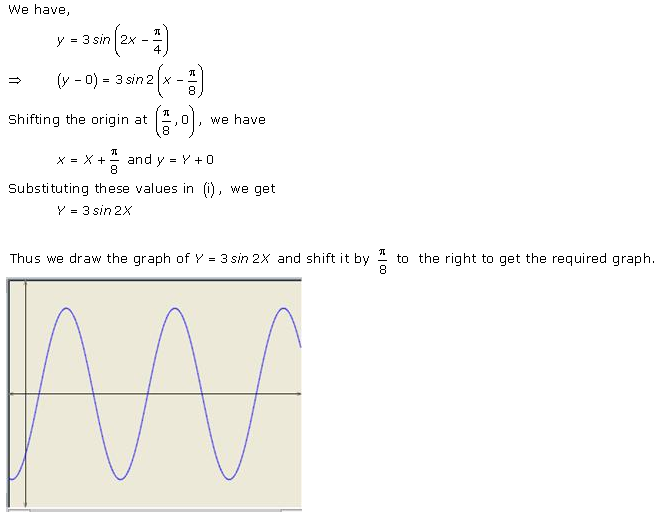RD Sharma Class 11 Solutions Chapter 6 Exercise 6.1 (Updated For 2021-22)RD Sharma Solutions Class 11 Maths Chapter 6 Exercise 6.1: Know more about Graphs of Trignometric functions in the RD Sharma Solutions Class 11 Maths Chapter 6 Exercise 6.1. To improve the performance of students in class 11 exams, we have designed RD Sharma class 11 solution for mathematics. You can download the PDF for free and start your exam preparation.

Download RD Sharma Solutions Class 11 Maths Chapter 6 Exercise 6.1 PDF:

RD Sharma Class 11 Solutions Chapter 6 Exercise 6.1

Access answers of RD Sharma Solutions for Class 11 Maths Chapter 6 Exercise 6.1

Chapter 6 Graphs of Trigonometric Functions Ex 6.1 Q1Chapter 6 Graphs of Trigonometric Functions Ex 6.1 Q2This is the complete blog of RD Sharma Solutions for Class 11 Maths Chapter 6 Exercise 6.1. To Know more about the CBSE Class 11 Maths exam, ask in the comments.

FAQs of RD Sharma Solutions for Class 11 Maths Chapter 6 Exercise 6.1

How many questions are there in RD Sharma Solutions for Class 11 Maths Chapter 6 Exercise 6.1?

There are 2 questions in RD Sharma Solutions Class 11 Maths Chapter 6 Exercise 6.1.